﻿ 机器学习(三)：理解逻辑回归及二分类、多分类代码实践_其他_开心洋葱网
• 欢迎访问开心洋葱网站，在线教程，推荐使用最新版火狐浏览器和Chrome浏览器访问本网站，欢迎加入开心洋葱 QQ群
• 为方便开心洋葱网用户，开心洋葱官网已经开启复制功能！
• 欢迎访问开心洋葱网站，手机也能访问哦~欢迎加入开心洋葱多维思维学习平台 QQ群
• 如果您觉得本站非常有看点，那么赶紧使用Ctrl+D 收藏开心洋葱吧~~~~~~~~~~~~~！
• 由于近期流量激增，小站的ECS没能经的起亲们的访问，本站依然没有盈利，如果各位看如果觉着文字不错，还请看官给小站打个赏~~~~~~~~~~~~~！

2379次浏览

# 一、逻辑回归：二分类

## 1.1 理解逻辑回归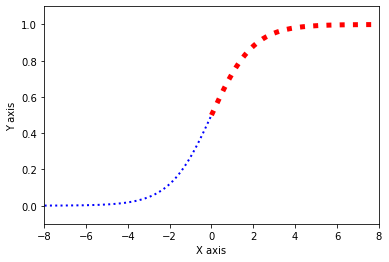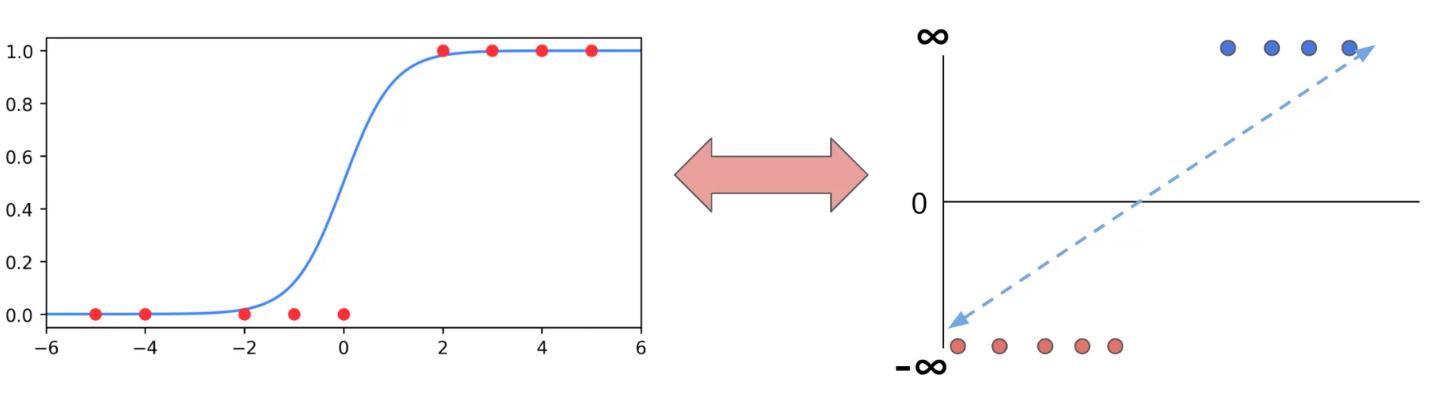$$\sigma(x) = \frac{1}{1+e^{-x}}$$

## 1.2 代码实践 – 导入数据集

import numpy as np
import pandas as pd
import seaborn as sns
import matplotlib.pyplot as plt


df = pd.read_csv('https://blog.caiyongji.com/assets/hearing_test.csv')

age physical_score test_result
33 40.7 1
50 37.2 1
52 24.7 0
56 31 0
35 42.9 1

• 特征：1. 年龄 2. 健康得分
• 标签：（1通过/0不通过）

## 1.3 观察数据

sns.scatterplot(x='age',y='physical_score',data=df,hue='test_result')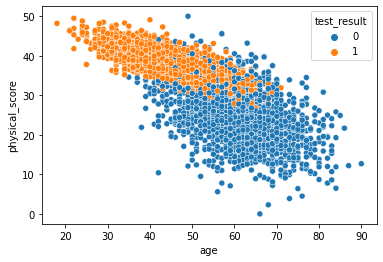sns.pairplot(df,hue='test_result')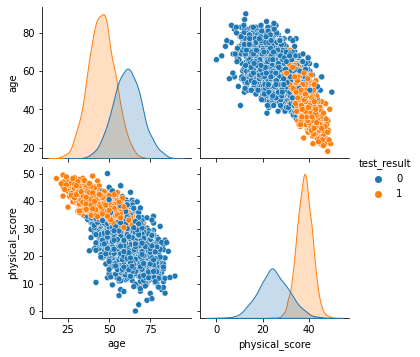## 1.4 训练模型

from sklearn.model_selection import train_test_split
from sklearn.preprocessing import StandardScaler
from sklearn.linear_model import LogisticRegression
from sklearn.metrics import accuracy_score,classification_report,plot_confusion_matrix

#准备数据
X = df.drop('test_result',axis=1)
y = df['test_result']
X_train, X_test, y_train, y_test = train_test_split(X, y, test_size=0.1, random_state=50)
scaler = StandardScaler()
scaled_X_train = scaler.fit_transform(X_train)
scaled_X_test = scaler.transform(X_test)

#定义模型
log_model = LogisticRegression()

#训练模型
log_model.fit(scaled_X_train,y_train)

#预测数据
y_pred = log_model.predict(scaled_X_test)
accuracy_score(y_test,y_pred)


# 二、模型性能评估：准确率、精确度、召回率

plot_confusion_matrix(log_model,scaled_X_test,y_test)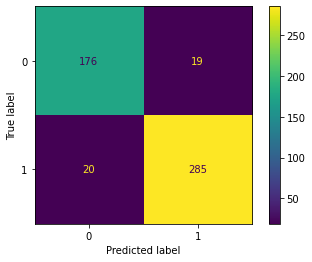• 真正类TP(True Positive) ：预测为正，实际结果为正。如，上图右下角285。
• 真负类TN(True Negative) ：预测为负，实际结果为负。如，上图左上角176。
• 假正类FP(False Positive) ：预测为正，实际结果为负。如，上图左下角19。
• 假负类FN(False Negative) ：预测为负，实际结果为正。如，上图右上角20。

$$Accuracy = \frac{TP+TN}{TP+TN+FP+FN}$$

$$Accuracy = \frac{285+176}{285+176+20+19} = 0.922$$

$$Precision = \frac{TP}{TP+FP}$$

$$Precision = \frac{285}{285+19} = 0.9375$$

$$Recall = \frac{TP}{TP+FN}$$

$$Recall = \frac{285}{285+20} = 0.934$$

print(classification_report(y_test,y_pred))# 三、Softmax：多分类

## 3.1 理解softmax多元逻辑回归

Logistic回归和Softmax回归都是基于线性回归的分类模型，两者无本质区别，都是从伯努利分结合最大对数似然估计。

Softmax回归模型首先计算出每个类的分数，然后对这些分数应用softmax函数，估计每个类的概率。我们预测具有最高估计概率的类，简单来说就是找得分最高的类。

## 3.2 代码实践 – 导入数据集

df = pd.read_csv('https://blog.caiyongji.com/assets/iris.csv')

sepal_length sepal_width petal_length petal_width species
5.1 3.5 1.4 0.2 setosa
4.9 3 1.4 0.2 setosa
4.7 3.2 1.3 0.2 setosa
4.6 3.1 1.5 0.2 setosa
5 3.6 1.4 0.2 setosa

• 特征：1. 花萼长度 2. 花萼宽度 3. 花瓣长度 4 花萼宽度
• 标签：种类：山鸢尾(setosa)、变色鸢尾(versicolor)和维吉尼亚鸢尾(virginica)

## 3.3 观察数据

sns.scatterplot(x='sepal_length',y='sepal_width',data=df,hue='species')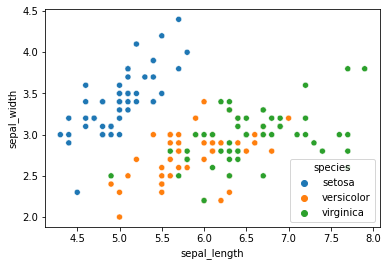sns.scatterplot(x='petal_length',y='petal_width',data=df,hue='species')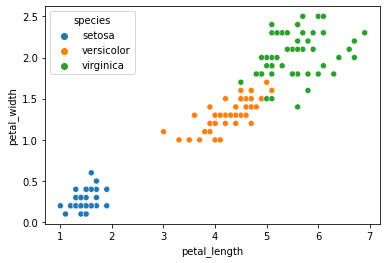sns.pairplot(df,hue='species')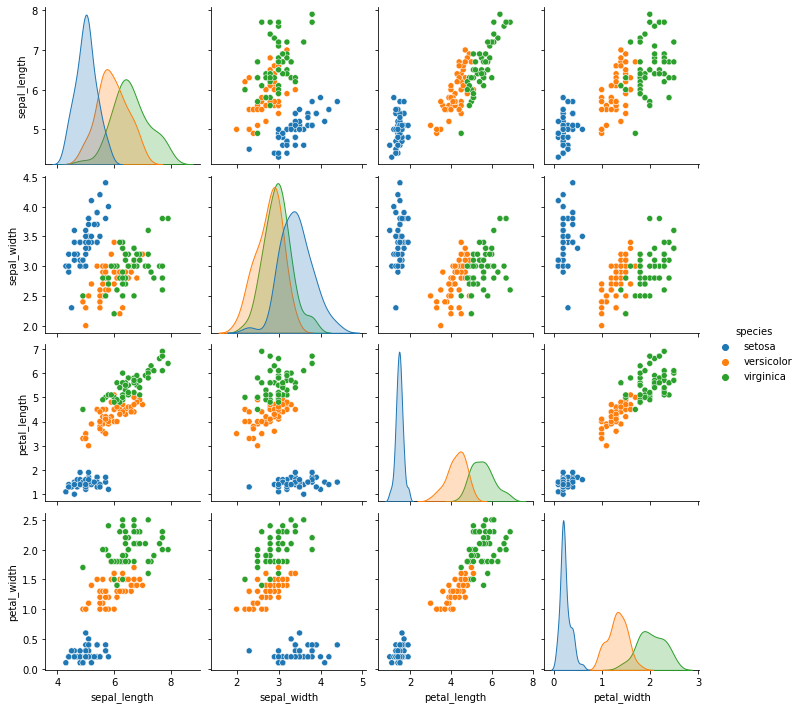## 3.4 训练模型

#准备数据
X = df.drop('species',axis=1)
y = df['species']
X_train, X_test, y_train, y_test = train_test_split(X, y, test_size=0.25, random_state=50)
scaler = StandardScaler()
scaled_X_train = scaler.fit_transform(X_train)
scaled_X_test = scaler.transform(X_test)

#定义模型
softmax_model = LogisticRegression(multi_class="multinomial",solver="lbfgs", C=10, random_state=50)

#训练模型
softmax_model.fit(scaled_X_train,y_train)

#预测数据
y_pred = softmax_model.predict(scaled_X_test)
accuracy_score(y_test,y_pred)


print(classification_report(y_test,y_pred))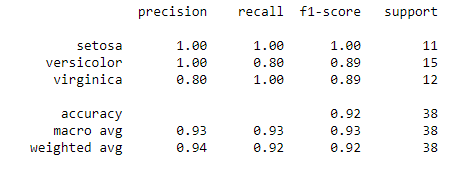## 3.5 拓展：绘制花瓣分类

#提取特征
X = df[['petal_length','petal_width']].to_numpy()
y = df["species"].factorize(['setosa', 'versicolor','virginica'])

#定义模型
softmax_reg = LogisticRegression(multi_class="multinomial",solver="lbfgs", C=10, random_state=50)

#训练模型
softmax_reg.fit(X, y)

#随机测试数据
x0, x1 = np.meshgrid(
np.linspace(0, 8, 500).reshape(-1, 1),
np.linspace(0, 3.5, 200).reshape(-1, 1),
)
X_new = np.c_[x0.ravel(), x1.ravel()]

#预测
y_proba = softmax_reg.predict_proba(X_new)
y_predict = softmax_reg.predict(X_new)

#绘制图像
zz1 = y_proba[:, 1].reshape(x0.shape)
zz = y_predict.reshape(x0.shape)
plt.figure(figsize=(10, 4))
plt.plot(X[y==2, 0], X[y==2, 1], "g^", label="Iris virginica")
plt.plot(X[y==1, 0], X[y==1, 1], "bs", label="Iris versicolor")
plt.plot(X[y==0, 0], X[y==0, 1], "yo", label="Iris setosa")
from matplotlib.colors import ListedColormap
custom_cmap = ListedColormap(['#fafab0','#9898ff','#a0faa0'])
plt.contourf(x0, x1, zz, cmap=custom_cmap)
contour = plt.contour(x0, x1, zz1, cmap=plt.cm.brg)
plt.clabel(contour, inline=1, fontsize=12)
plt.xlabel("Petal length", fontsize=14)
plt.ylabel("Petal width", fontsize=14)
plt.legend(loc="center left", fontsize=14)
plt.axis([0, 7, 0, 3.5])
plt.show()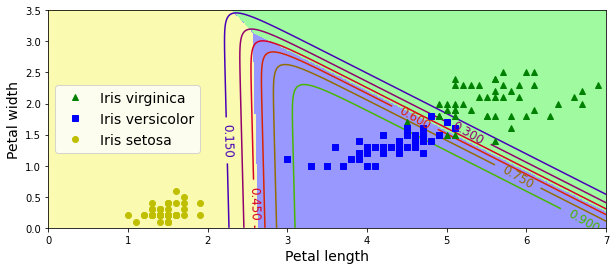# 四、小结

1. 机器学习的分类
2. 机器学习的工业化流程
3. 特征、标签、实例、模型的概念
4. 过拟合、欠拟合
5. 损失函数、最小二乘法
6. 梯度下降、学习率
7.线性回归、逻辑回归、多项式回归、逐步回归、岭回归、套索(Lasso)回归、弹性网络(ElasticNet)回归是最常用的回归技术
7. Sigmoid函数、Softmax函数、最大似然估计

• 机器学习(二)：理解线性回归与梯度下降并做简单预测
• 机器学习(一)：5分钟理解机器学习并上手实践
• 前置机器学习（五）：30分钟掌握常用Matplotlib用法
• 前置机器学习（四）：一文掌握Pandas用法
• 前置机器学习（三）：30分钟掌握常用NumPy用法
• 前置机器学习（二）：30分钟掌握常用Jupyter Notebook用法
• 前置机器学习（一）：数学符号及希腊字母

• 版权声明

本站的文章和资源来自互联网或者站长
的原创，按照 CC BY -NC -SA 3.0 CN
协议发布和共享，转载或引用本站文章
应遵循相同协议。如果有侵犯版权的资
源请尽快联系站长，我们会在24h内删
除有争议的资源。
• 合作网站

• 友情链接

• 关于我们

一群热爱思考，热爱生活，有理想的新社会主义接班人的多维思维学习平台，天行健，君子以自强不息。地势坤，君子以厚德载物。